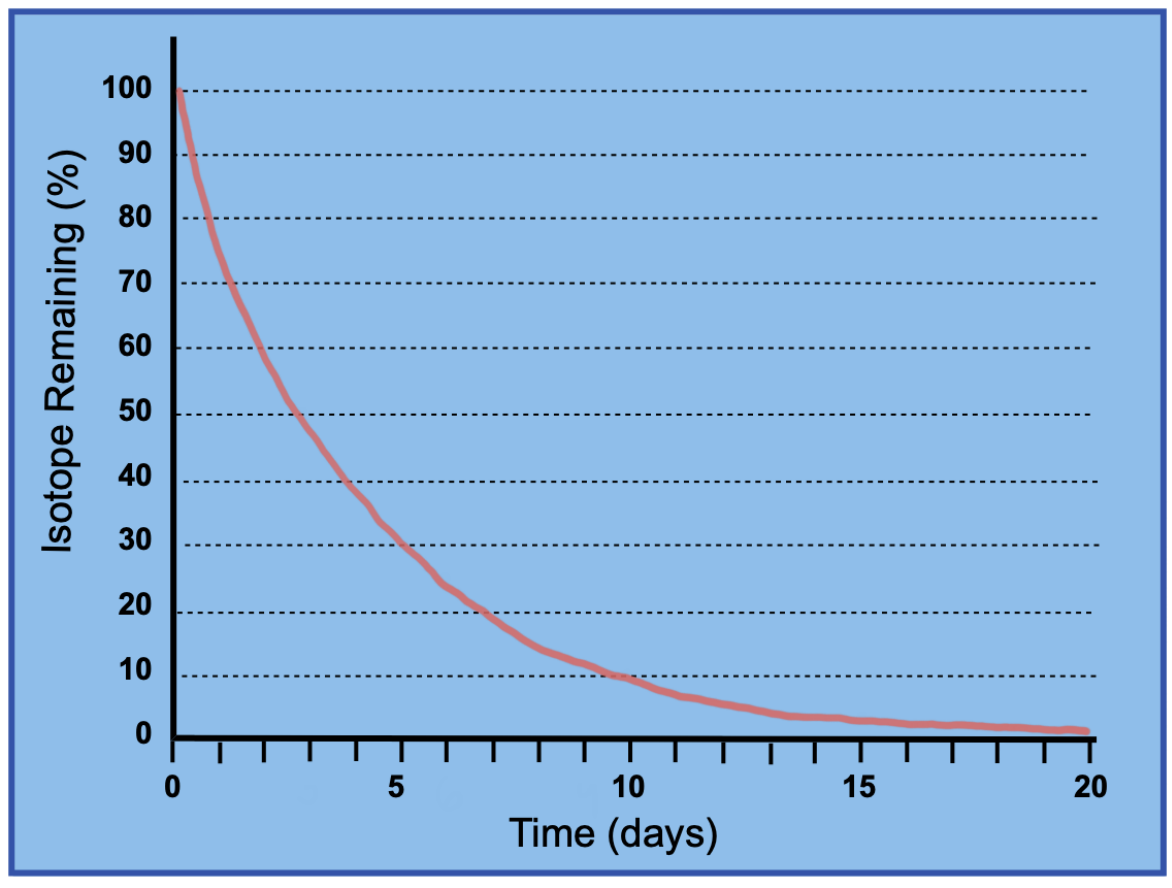Clutch Prep is now a part of Pearson
Ch.11 Nuclear ChemistryWorksheetSee all chapters

See all sections
Sections
Alpha Decay
Beta Decay
Gamma Emission
Electron Capture
Positron Emission

Half-Life is the amount of time required for half of a radioisotope to decay.

###### Calculating Radioactive Half-Life

Concept #1: Understanding Radioactive Decay

Concept #2: Radioisotope Remaining

Example #1: Sodium-24, a radioisotope used in examining human circulation, has a half-life of around 15 days. Approximately what percentage of a sample would remain after 3 months, if a month is considered 30 days?

Practice: The half-life of arsenic-74 is about 18 days. If a sample initially contains 100.00 mg arsenic-74, what mass (in mg) would be left after 72 days?

Practice: The half-life of iodine-131, an isotope used in thyroid therapy, is 8.021 days. What percentage of iodine-131 remains in a sample that is estimated to be 40.11 days old?

Practice: What is the concentration of a CO2 in a container after 4 half-lives if 0.325 mol of CO2 is initially placed into a 5.0 L reaction vessel?

Practice: What is the half-life of the radioisotope that shows the following plot of remaining percentage vs. time?